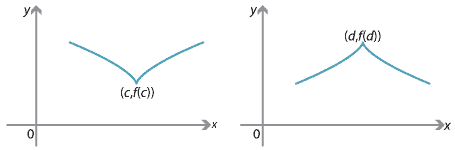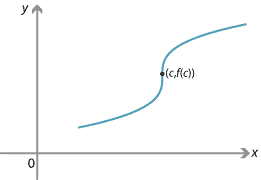### Critical points

In the section Graph sketching, we looked at stationary points of a function $$f$$, that is, points where $$f'(x)=0$$. We gave conditions for a stationary point to be local minimum or a local maximum.

Local maxima and minima also occur in other cases. The point $$(c,f(c))$$ on the left-hand graph is a local minimum. The point $$(d,f(d))$$ on the right-hand graph is a local maximum. These are examples of critical points. In each case, the function is not differentiable at that point.Figure : Examples of critical points.

###### Definition

A critical point for a function $$f$$ is any value of $$x$$ in the domain of $$f$$ at which $$f'(x) = 0$$ or at which $$f$$ is not differentiable.

Every stationary point is a critical point.

Not every critical point is a local minimum or maximum point. In the following diagram, the function is not differentiable at the point $$(c,f(c))$$, since the tangent is vertical. There is a point of inflexion at $$(c,f(c))$$.Next page - Links forward - Finding gradients on a parametric curve